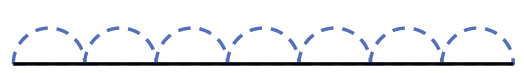### Home > CC1 > Chapter 4 > Lesson 4.1.1 > Problem4-7

4-7.

Croakie now has a new routine that is $59$ feet long. Keep this distance in mind as you complete parts (a) and (b) below. Homework Help ✎

1. In his new routine, Croakie makes seven super jumps, all the same length, and then hops $3$ feet. How long is each super jump?

A diagram like the one below should help.Before Croakie had to hop 3 feet, how far had he jumped?

If Croakie made $7$ jumps that were all the same length to go 56 feet, how long was each jump?

$59\ \text{feet}−3\text{ feet}=56\text{ feet}$

$\frac{56}{7}=8$

$8$ feet per jump

1. If x represents the length of one super jump and $2x$ represents the length of two super jumps, write an expression that represents Croakie’s routine.

If you did $3$ super jumps and then walked 5 feet the expression would be $3x + 5$.